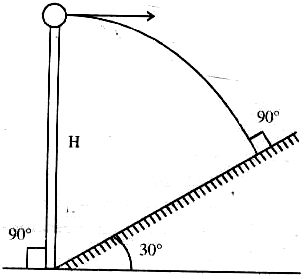# The answer to each of the questions is a single digit integer ranging from 0 to 9.In the given figure, the angle of inclination of the inclined plane is 30o. Find the horizontal velocity v0 (in m/s) so that the particle hits the inclined plane perpendicularly. Given h = 4m, g = 10 m/s2.9 years ago

4

q = v0cos 30o – g sin 30o t Þ t =                    …… (1)

– H cos 30o = –v0 sin 30o t –  g cos 30ot2                …… (2)

By equation (1) and (2), we get

H =

= 4 m/s